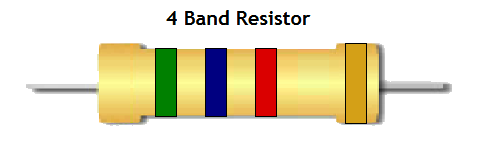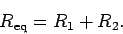# How does a Resistor Work | Resistor Color Code | Application of Resistors

## ResistorA resistor is a passive two terminal electrical component that implements electrical resistance as a circuit element. In electronic circuits, resistors are used to reduce current flow, adjust signal levels, to divide voltages, bias active elements, and terminate transmission line, among other uses.  High-power resistors that can dissipate many watts of electrical power as heat. It may be used as part of motor controls, in power distribution systems, or as test loads for generators.  Fixed resistors have resistances that only change slightly with temperature, time or operating voltage.It has most important function is discrete components can be composed of various compounds and forms. Resistors are also implemented with in integrated circuit.

Resistor color codinga) Band 1 is the first significant figure of component value (left side) of the resistor. b) Band 2 is the second significant figure (some precision resistors have a third significant figure, and thus five bands). c) Band 3 is the decimal multiplier. d) Band 4 if present, indicates tolerance of value in percent (no band means 20%).Learn about of Resistor Color Code in one minute-:  B.B  R.O.Y  Great Britisian Very Good Wife (This word is God gift for you).

Means of this word is -:

BB(BLACK-BROWN) Roy(Red-Orange-Yellow) Great(Green) Britisian(Blue) Very(Violet) Good(Gray) Wife(White).

We can consider two process fo resistance measured(i) Parallel Method. (ii) Series Method.

(i) Parallel Method

Here three resistor (R1,R2,R3) all are Parallel connection.This law is applicable only for when all resistor are Parallel connection.

(ii) Series Method

Here three resistor (R1,R2,R3) all are Series connection.This law is applicable only for when all resistor are Series connection.

Variable ResistorWhat is Variable Resistor-: A variable resistor is in essence an electro-mechanical transducer and normally works by sliding a contact (wiper) over a resistive element.  It can be used as a three terminal as well as a two terminal device. Mostly they are used as a three terminal device.Variable resistors are mostly used for device calibration.

Application of Variable Resistors

There are mainly three types of variable resistors Are occurs:- (i) Potentiometer (ii) Rheostat (iii) Presets

(i) PotentiometerWhat is Potentiometer-: A potentiometer is a three-terminal resistor with a sliding or rotating contact that forms an voltage devider. If only two terminals are used, one end and the wiper, it acts as a variable resistor.

(ii) RheostatWhat is Rheostat-: Rheostat are very similar in construction to potentiometers. But are not used as a potential divider. Its behavior like a variable resistance. They use only two terminals instead of the 3 terminals potentiometers use. One connection is made at one end of the resistive element, the other at the wiper of the variable resistor. Where the rheostat must be rated for higher power (more than about 1 watt). It may be built with a resistance wire wound around a semicircular insulator, with the wiper sliding from one turn of the wire to the next. Sometimes a rheostat is made from resistance wire wound on a heat-resisting cylinder, with the slider made from a number of metal fingers that grip lightly onto a small portion of the turns of resistance wire. The “fingers” can be moved along the coil of resistance wire by a sliding knob thus changing the “tapping” point. Wire-wound rheostats made with ratings up to several thousand watts are used in applications such as DC motor drives, electric welding controls, or in the controls for generators.

(iii) PresetsWhat is Presets-: A preset  is a smaller PCB mounted version of a potentiometer. They can be placed easily on a PCB and can also be adjustable when needed. The value of the resistance is normally adjusted with the help of a screw-driver. They are frequently used in applications that have adjustable frequency tone of the alarm or adjustable sensitivity circuits. These are also highly specific presets that have multi-turn options. In this type of presets, the resistances are increased or decreased gradually and therefore the screw has to be rotated more number of times.